December 2019## The Presence of Dependence

• How might we represent dependence
• Correlation
• Recall “Tobler’s Law”
• Correlation as a function of “nearness”
• Represent nearness for different kinds of spatial data
• Points - distance

• Conditional expected value of an area depends on adjacent area values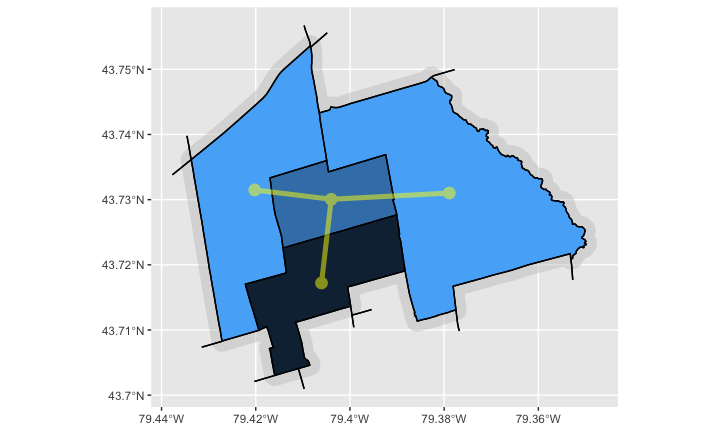## Further Details

• One possible model is:

$\left( x_i \ | \ x_1, \cdots , x_{i-1}, \cdots , x_n \right) \sim N \left( \sum_j w_{ij} x_j, \tau^2 \right)$

• here conditional distribution of $$x_i$$ is Gaussian
• Mean is the mean of its neighbours
• Variance is $$\tau^2$$
• Defines the smoothness of the pattern
• The specification is ambigous
• No change to densities if you add a constant to all $$x_i$$
• Resolve by specifying $$E(x_i) = 0$$ for joint distribution
• Named CAR (Conditional AutoRegressive) model
• An example of a Markov Random Field (MRF)

## Example - Irish counties mean age data

age_cty <- st_read('ages.json')
• Here we model $$x_i$$ with a CAR model, plus a constant mean added on
• $$\mathbf{X} = \mu + \mathbf{S}$$
• $$\mathbf{S} \sim \textsf{CAR}(\tau^2)$$
• $$\mu$$ is constant overall mean of each $$x_i$$ in $$\mathbf{X}$$
• We use package mgcv to model this
• CAR is specified by s(COUNTY,bs='mrf',nb=adj_list)
• adj_list is a county adjacency list
• but mgcv requires a named list with the county names
• predictreturns fitted values for $$x_i$$’s
library(mgcv)
names(adj_list) <- age_cty$COUNTY CAR_model <- gam(Mean_Age ~ s(COUNTY, xt=list(nb=adj_list),bs='mrf'),data=age_cty, method='REML') age_cty$fitted <- predict(CAR_model)
ggplot(age_cty,aes(fill=fitted)) + geom_sf(lwd=0.2) +
scale_fill_viridis_c(name='Fitted\nValue',direction=-1)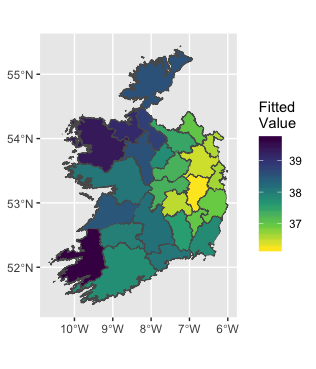## Further investigation

summary(CAR_model)
Family: gaussian

Formula:
Mean_Age ~ s(COUNTY, xt = list(nb = adj_list), bs = "mrf")

Parametric coefficients:
Estimate Std. Error t value Pr(>|t|)
(Intercept)  37.7259     0.1642   229.8   <2e-16 ***
---
Signif. codes:  0 '***' 0.001 '**' 0.01 '*' 0.05 '.' 0.1 ' ' 1

Approximate significance of smooth terms:
edf Ref.df     F p-value
s(COUNTY) 12.58     25 1.771  0.0116 *
---
Signif. codes:  0 '***' 0.001 '**' 0.01 '*' 0.05 '.' 0.1 ' ' 1

R-sq.(adj) =  0.639   Deviance explained = 82.1%
-REML = 43.302  Scale est. = 0.70083   n = 26
AIC(CAR_model)
 84.62551
ggplot(age_cty,aes(x=Mean_Age,y=fitted)) +
geom_point() +
geom_smooth(method=MASS::rlm,se = FALSE) + coord_equal()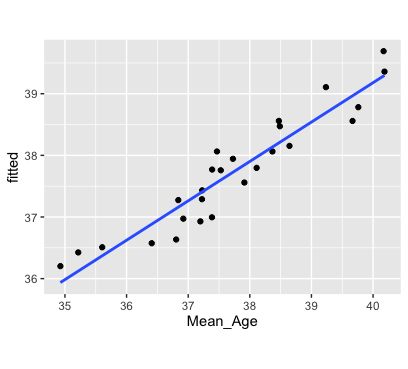## CAR Embedded in A Model - Broadband Uptake

• The data can be obtained through this link and is in an R data file named ireland_ct_ed.RData. Assuming you have downloaded this and put in into the R folder you are working with, load it using:
load('ireland_ct_ed.RData')
• its a set of sf objects
• ED_2016 Irish Enumeration Districts
• CTY_2016 Irish Counties (already have this)
colnames(ED_2016)
  "OBJECTID"             "ED_ID"                "ED_ENGLISH"           "ED_GAEILGE"           "COUNTY"               "CONTAE"
 "PROVINCE"             "CENTROID_X"           "CENTROID_Y"           "GUID_"                "CSOED_3409"           "OSIED_3441"
 "CSOED_34_1"           "Shape__Are"           "Shape__Len"           "GUID"                 "GEOGID"               "Age0_4"
 "Age5_14"              "Age25_44"             "Age45_64"             "Age65over"            "EU_National"          "ROW_National"
 "Born_outside_Ireland" "Separated"            "SinglePerson"         "Pensioner"            "LoneParent"           "DINK"
 "NonDependentKids"     "RentPublic"           "RentPrivate"          "Flats"                "NoCenHeat"            "RoomsHH"
 "PeopleRoom"           "SepticTank"           "HEQual"               "Employed"             "TwoCars"              "JTWPublic"
 "HomeWork"             "LLTI"                 "UnpaidCare"           "Students"             "Unemployed"           "EconInactFam"
 "Agric"                "Construction"         "Manufacturing"        "Commerce"             "Transport"            "Public"
 "Professional"         "Internet"             "Broadband"            "Broadband_Count"      "Broadband_Denom"      "geometry"            

## Further Details - Broadband Model

• Broadband The proportion of households with broadband access.
• Professional The proportion of economically active population employed in professional occupations.
• Age65over The proportion of the population aged 65 or over.
• COUNTY the name of the county that the ED is within. Note that EDs do not overlap county boundaries.
• Also Broadband_Count and Broadband_Denom
Broadband <- Broadband_Count / Broadband_Denom
ggplot(ED_2016,aes(fill=Broadband)) +
geom_sf(lwd=NA) +
scale_fill_viridis_c(name="Broadband\nPropn.")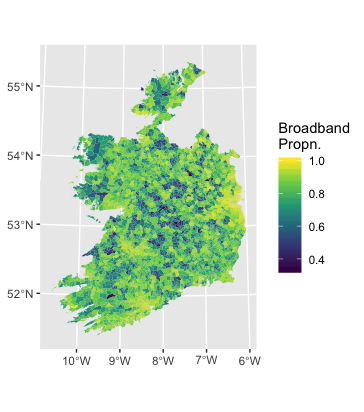## Binomial Model with Spatial Term

• $$\textsf{logit}(p_{BB}) = \alpha + \beta_1x_1 + \beta_2x_2 + \gamma_j$$
• $$\beta_1$$ - Professional
• $$\beta_2$$ - Age650ver
• $$\gamma_j$$ - effect of being in county $$j$$ $$\gamma_j$$ is CAR($$\tau^2$$)
• Also a multi-level model…
nlist <- CTY_2016 %>% st_touches()
names(nlist) <- CTY_2016$COUNTY bb_CAR <- gam(Broadband~Professional+Age65over + s(COUNTY,bs='mrf',xt=list(nb=nlist)), data=ED_2016, family=binomial, weight=Broadband_Denom, method='REML') summary(bb_CAR) Family: binomial Link function: logit Formula: Broadband ~ Professional + Age65over + s(COUNTY, bs = "mrf", xt = list(nb = nlist)) Parametric coefficients: Estimate Std. Error z value Pr(>|z|) (Intercept) 1.56010 0.02040 76.49 <2e-16 *** Professional 3.05310 0.07930 38.50 <2e-16 *** Age65over -1.82430 0.06355 -28.70 <2e-16 *** --- Signif. codes: 0 '***' 0.001 '**' 0.01 '*' 0.05 '.' 0.1 ' ' 1 Approximate significance of smooth terms: edf Ref.df Chi.sq p-value s(COUNTY) 24.77 25 22234 <2e-16 *** --- Signif. codes: 0 '***' 0.001 '**' 0.01 '*' 0.05 '.' 0.1 ' ' 1 R-sq.(adj) = 0.235 Deviance explained = 38.6% -REML = 33031 Scale est. = 1 n = 3409 ## Maps of Spatial Effects county_extract <- tibble(Professional=rep(0,26), Age65over=rep(0,26), COUNTY=levels(CTY_2016$COUNTY))
CTY_2016<- CTY_2016 %>% mutate(agamma_i=predict(bb_CAR,
newdata = county_extract))
ggplot(CTY_2016,aes(fill=agamma_i)) + geom_sf() +
scale_fill_distiller(name=expression(alpha+gamma[i]))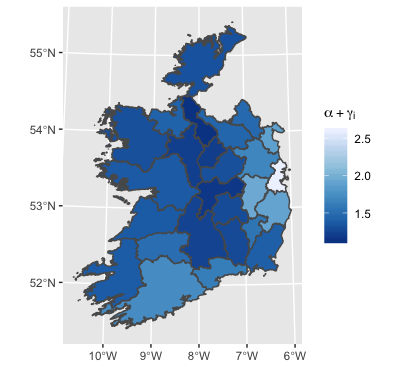CTY_2016<- CTY_2016 %>%
mutate(gamma_i=predict(bb_CAR,
newdata = county_extract,type='terms')[,3])
ggplot(CTY_2016,aes(fill=gamma_i)) + geom_sf() +
scale_fill_distiller(type='div',
name=expression(gamma[i]),
limits=c(-1.2,1.2))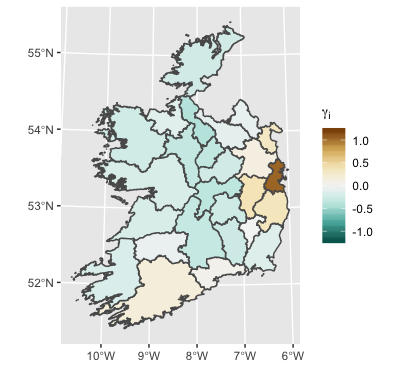## A Further Effect

• $$\textsf{logit}(p_{BB}) = \alpha + \beta_1x_1 + \beta_2x_2 + \gamma_{0j} + \gamma_{1j}x_1$$
• with $$\gamma_{0j} \sim \textsf{CAR}(\tau_0^2)$$ and $$\gamma_{1j} \sim \textsf{CAR}(\tau_1^2)$$
• Professional coefficient varies spatially
bb_CAR2 <- gam(Broadband~Professional+Age65over +
s(COUNTY,bs='mrf',xt=list(nb=nlist)) +
s(COUNTY,bs='mrf',xt=list(nb=nlist),
by=Professional,k=6),
data=ED_2016, family=binomial,
weight=Broadband_Denom, method='REML')
• Map on right
• Contrast between high and low proportion of Professionals really high in Dublin…
• N.B. $$k$$ is a smoothing parameter - low values cut out higher frequency variation in $$\gamma$$’s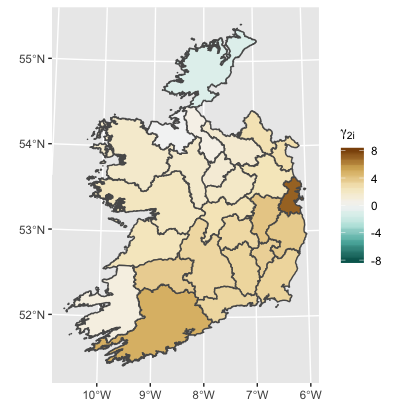## And finally…

• $$\textsf{logit}(p_{BB}) = \alpha + \beta_1x_1 + \beta_2x_2 + \gamma_{i}$$
• with $$\gamma_{i} \sim \textsf{CAR}(\tau^2)$$ but at ED level
• CAR process is at individual observation level
• Some islands were defined to be ‘adjacent’ to nearest land EDs.
• See map - do counties reflect pattern?
• MAUP (Modifiable Areal Unit Problem)